## IF Function

The IF function is a premade function in Google Sheets, which returns values based on a true or false condition.

It is typed `=IF` and has 3 parts:

=IF(logical_expression, value_if_true, value_if_false)

The condition is referred to as `logical_expression`, which can check things like:

• If a number is greater than another number `>`
• If a number is smaller than another number `<`
• If a number or text is equal to something `=`

Note: You can decide both the return values and the condition.

## Example IF function (equal to)

Check if the Pokemon type is grass:

The condition is if the "Type 1" value for the Pokemon is "Grass".

The function returns "Yes" or "No".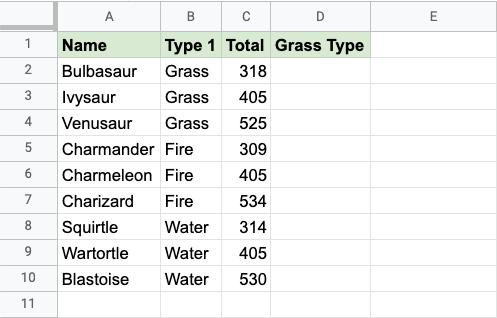Copy Values

Example IF function, step by step:

1. Select the cell `D2`
2. Type `=IF`
3. Click the IF command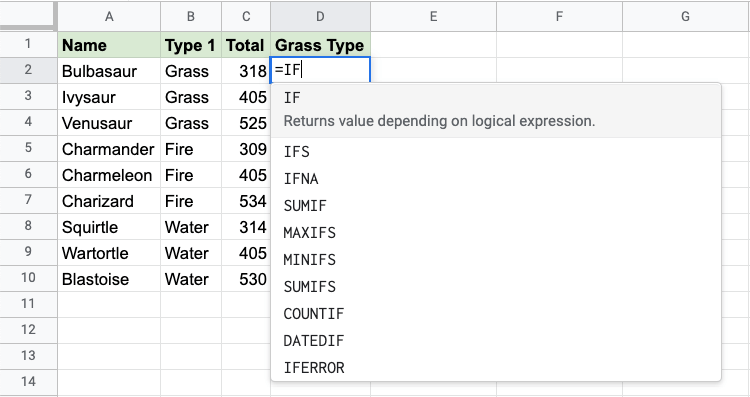1. Specify the condition `B2="Grass"`
2. Type `,`
3. Specify the value `"Yes"` for when the condition is TRUE
4. Type `,`
5. Specify the value `"No"` for when the condition is FALSE
6. Hit enter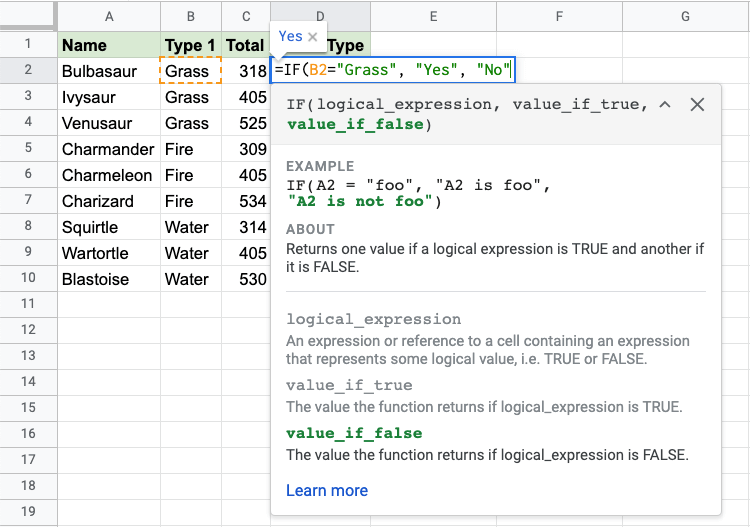Since the value in cell `B2` is "Grass", the condition is true and the function will return "Yes".

Note: Text values needs to be in quotes: " "

The function can be repeated with the filling function for each row to perform the same check for each Pokemon: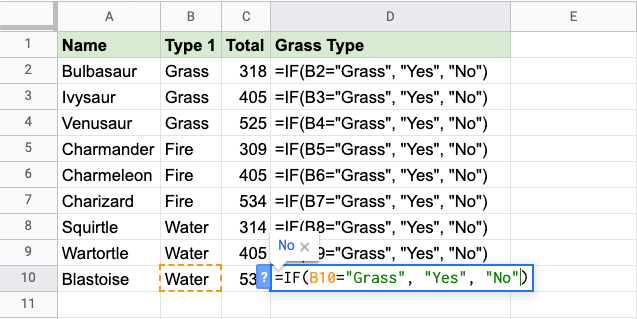Now, each row has a check for Grass Type: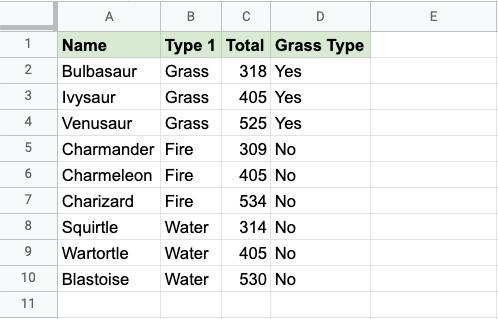## Example IF Function (greater than)

Check if the Pokemon's total stats is greater than 500:

The function returns "Yes" or "No".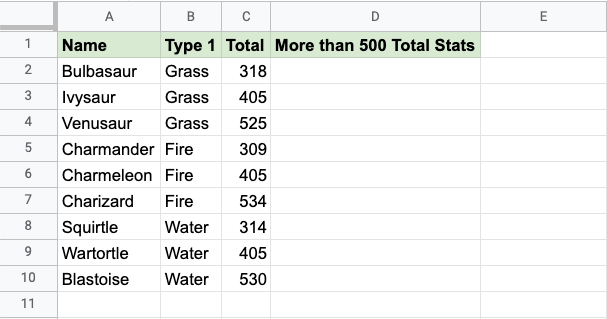Copy Values

Example IF function, step by step:

1. Select the cell `D2`
2. Type `=IF`
3. Click the IF command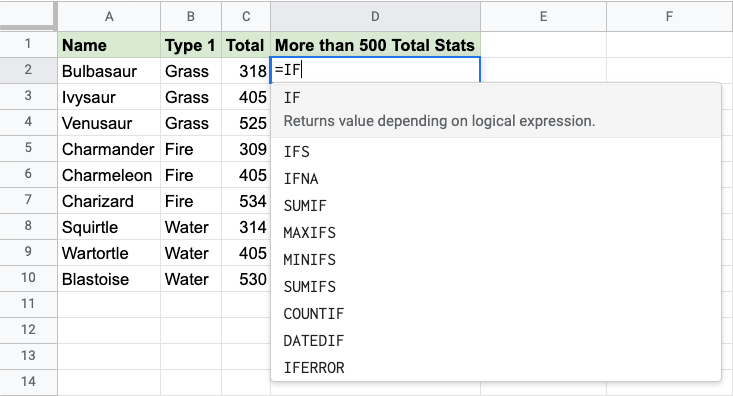1. Specify the condition `C2>500`
2. Type `,`
3. Specify the value `"Yes"` for when the condition is TRUE
4. Type `,`
5. Specify the value `"No"` for when the condition is FALSE
6. Hit enter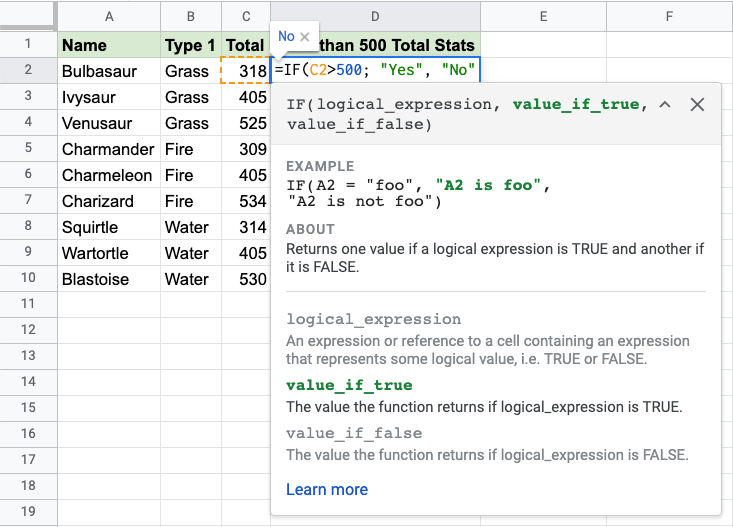Since the value in cell `C2` is 318, the condition is false and the function will return "No".

The function can be repeated with the filling function for each row to perform the same check for each Pokemon: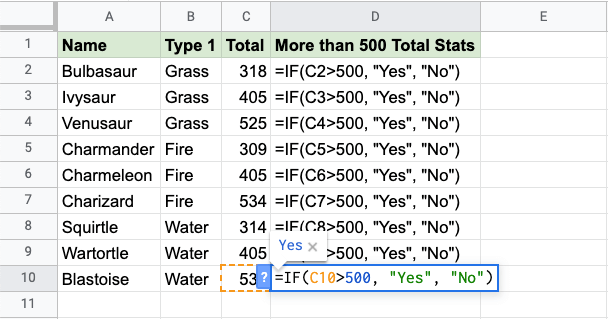Now, each row has a check for having more than 500 total stats: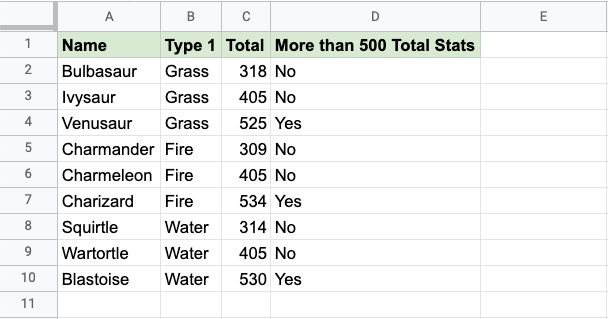W3Schools is optimized for learning and training. Examples might be simplified to improve reading and learning. Tutorials, references, and examples are constantly reviewed to avoid errors, but we cannot warrant full correctness of all content. While using W3Schools, you agree to have read and accepted our terms of use, cookie and privacy policy.